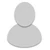## FANDOM

13,593 Pages

• So we're all familiar with Logic Manipulation, the ability to manipulate the very fundamentals of logic itself.

However, recently I have stumbled upon something called Metalogic, which is by definition "logic beyond logic".

Thus, I'd like to bring up the topic of Metalogic Manipulation.

How would it work? What would be the results of altering a uni/multi/omniversal structure's metalogic? Would it be a limitless version of Logic Manipulation, which in turn is a limitless version of Reality Warping?

• Metalogic is not 'logic beyond logic' - it is the logic of logic, the study of the actual structure and properties of logic, rather than the study of logical systems.

In other words, you are using logic to study logic itself, rather than a specific subject, argument, theory, etc.

Metalogic manipulation is still logic manipulation.

Another example is metamathematics, which is using mathematics to talk about mathematics.

It's all meta. "Meta" literally means "referring to itself."

Related: I'm So Meta, Even This Acronym

•